# President's Update

HCBA/TKA Alumni,

Greetings from 202 Smothers Road! I pray that each of you are doing well! Our last alumni update you received from last quarter was a picture of all that the Lord did through the previous academic year. I hope that you enjoyed the opportunity to see how the Lord has moved in such mighty ways.

This newsletter is intended to provide highlights on what is occurring on campus, opportunities for alumni involvement, and ways that you can pray and support the efforts of The King's Academy.

I am pleased to report that TKA has been blessed with an 11% enrollment increase at the start school year, and despite dealing with the pandemic, the school has seen a very successful year in our classrooms, fields of competition, etc. The heart of our leadership team, faculty, and staff is to develop servant leaders that will change the world for Christ. We are excited to see students from the following nations join our Lion family: Aruba, Brazil, Czech Republic, China, Croatia, Curacao, Hong Kong, Italy, Japan, Montenegro, Rwanda, and Uganda.

I have fully enjoyed the opportunity to get to know many of our esteemed alumni and cherish the stories and impacts that are shared with me on a weekly basis. I would like to personally invite you to participate in an upcoming alumni activity/event or to drop by campus when you are in the area. I look forward to getting to know as many of you as possible in the days and weeks ahead.

In Christ,

Jeremy Sandefur
President

 table div table+table+table div table{width:100%;padding:0}table div table+table+table div table img{width:96.23%;padding:0;float:none}table div table+table+table div table td{width:100%;padding:0 1.88% 18px}/* styles */# Homecoming 2022 Events

## Friday, October 21

Save the date of October 21st for Homecoming 2022! All alumni are encouraged to join us as we celebrate 143 years of faith-based education.

Any classes who would like to host a reunion during Homecoming 2022, please notify Lori Campbell (alumni@thekingsacademy.net) for assistance.# Homecoming Parking

Parking for Alumni will be reserved in the Tibbetts parking lot. When you arrive on campus, please park in this area. You will not need to move your car for the parade, pep rally, tailgating, or game.

The cost of parking is included in the the Homecoming ticket package that can be purchased on the link above.## 1:30 p.m.

Join us for our homecoming parade at 1:30 p.m. in front of Anderson, and stay for the pep rally immediately following on Huskey field.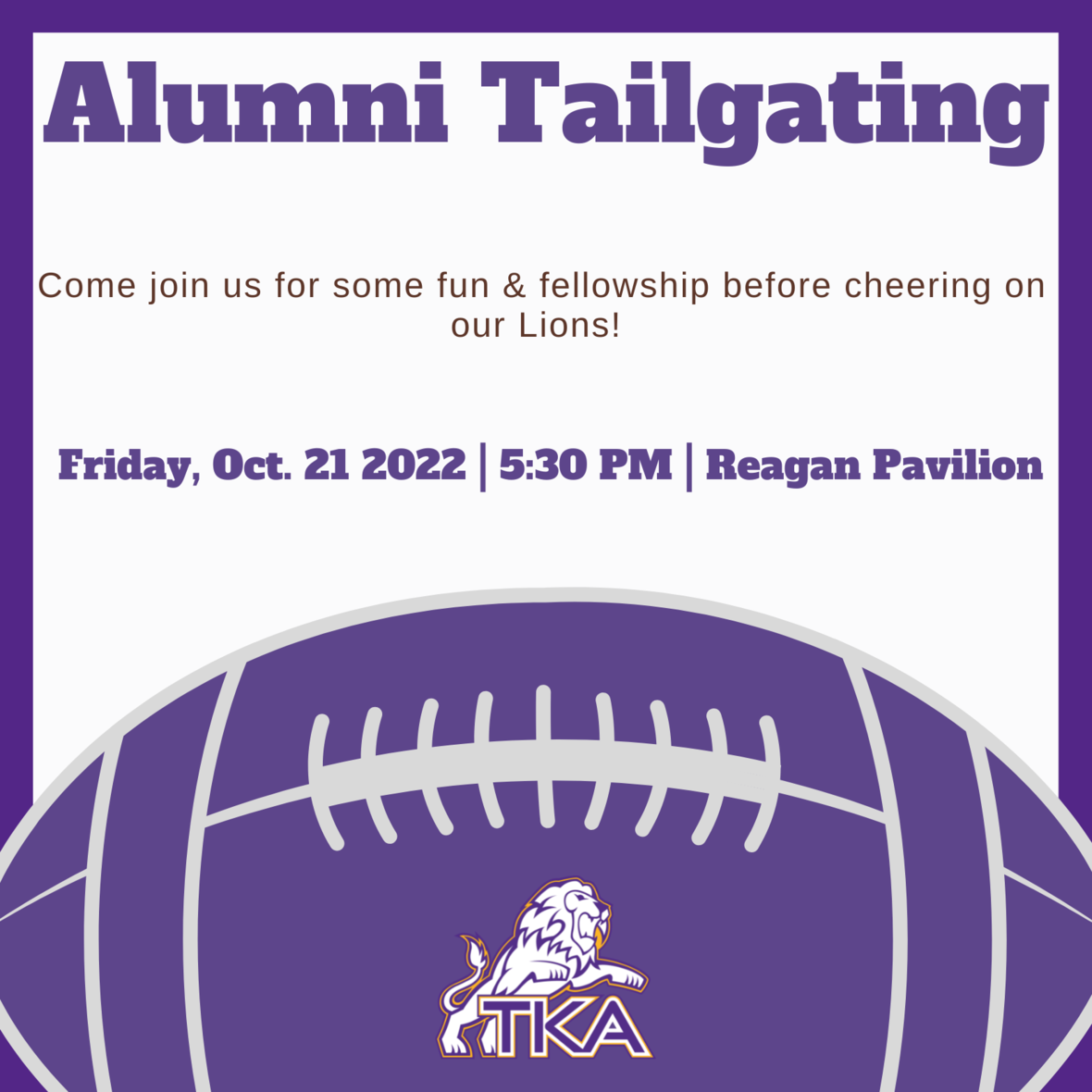# Alumni Tailgate

## 5:30 p.m.

The King's Academy will host an Alumni tailgate at the Reagan Pavilion. Please purchase tickets by using the link below. Tickets include: discounted game tickets, parking, and tailgating meal. Tickets are \$20 for adults, \$10 for students 6-17, and students under 5 are free. We look forward to seeing all of our alumni at this event!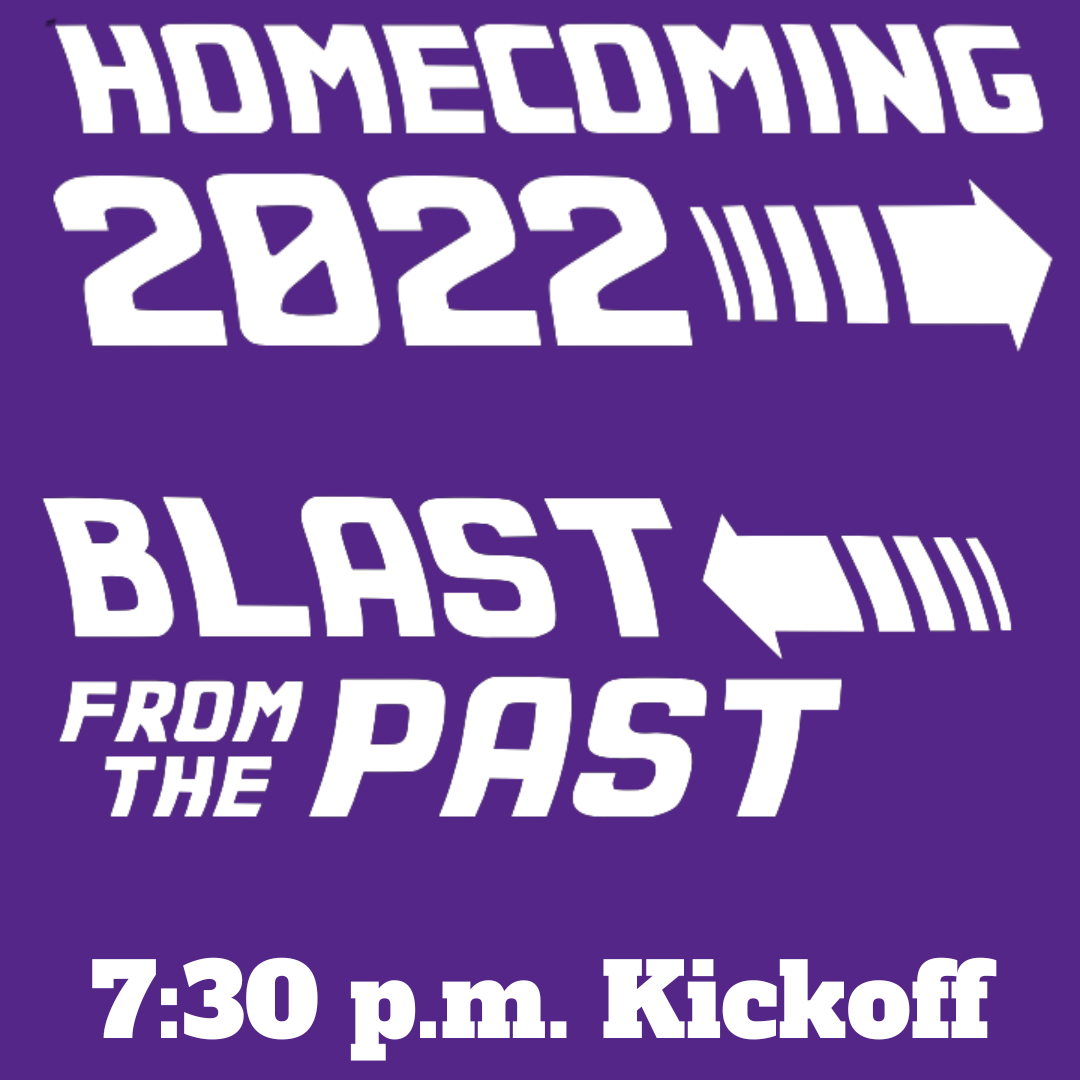# Game Time

▪ 7:00 p.m. Presentation of 2022 Homecoming Court
▪ 7:30 p.m. Kickoff against Webb Bell Buckle
 ▪ 7:00 p.m. Presentation of 2022 Homecoming Court
 ▪ 7:30 p.m. Kickoff against Webb Bell Buckle

Alumni can sit in the stands at the game or bring lawn chairs to sit on the side near the stone wall.# School Store

The Lion's Den (school store) will have Academy merchandise for sale at the Homecoming game. It will be located near the Concession Stand at Stokley.

 table div table+table+table+table+table+table+table+table+table+table+table+table+table div table{width:100%;padding:0}table div table+table+table+table+table+table+table+table+table+table+table+table+table div table img{width:96.23%;padding:0;float:none}table div table+table+table+table+table+table+table+table+table+table+table+table+table div table td{width:100%;padding:0 1.88% 18px}/* styles */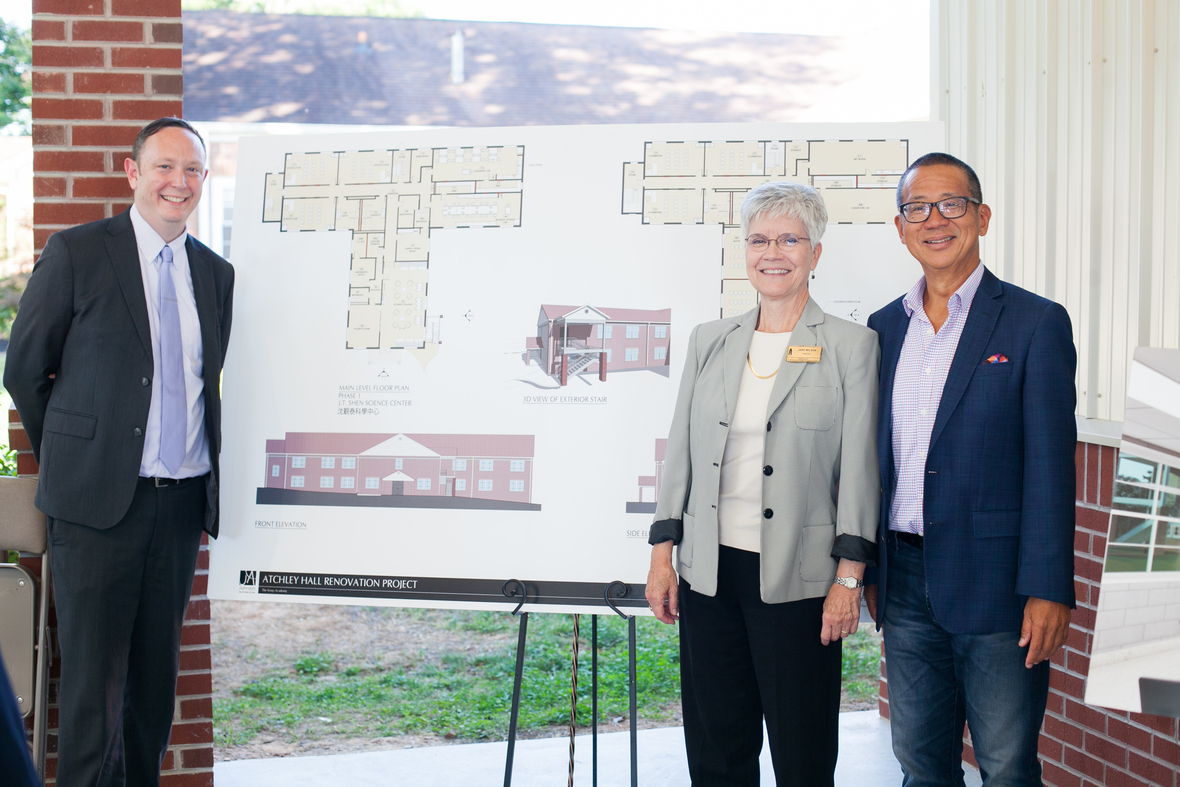# J.T. Shen Science Center at Atchley Hall Dedication

We are thankful for all that God is doing here at The Academy! We recently had our dedication ceremony for the J.T. Shen Science Center at Atchley Hall. We are thankful for all of our donors that have made this first step in renovation possible.

Take a moment and look over some of our exciting renderings and pictures from the dedication ceremony.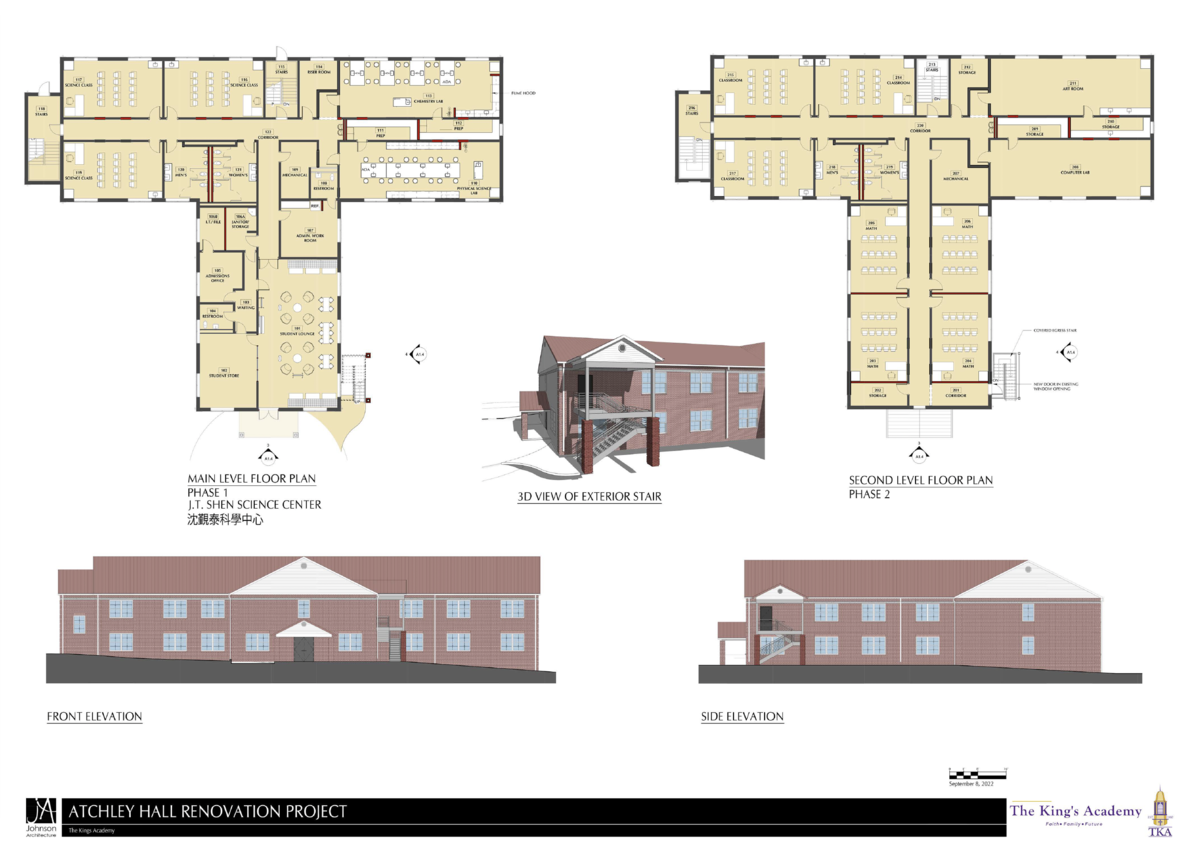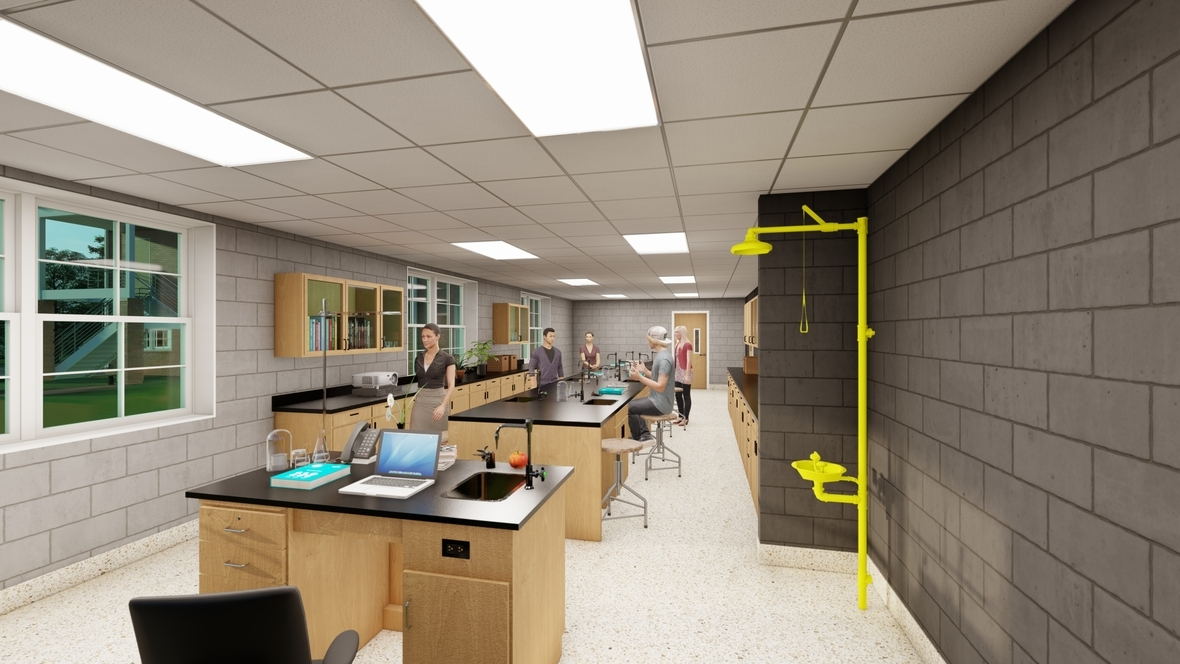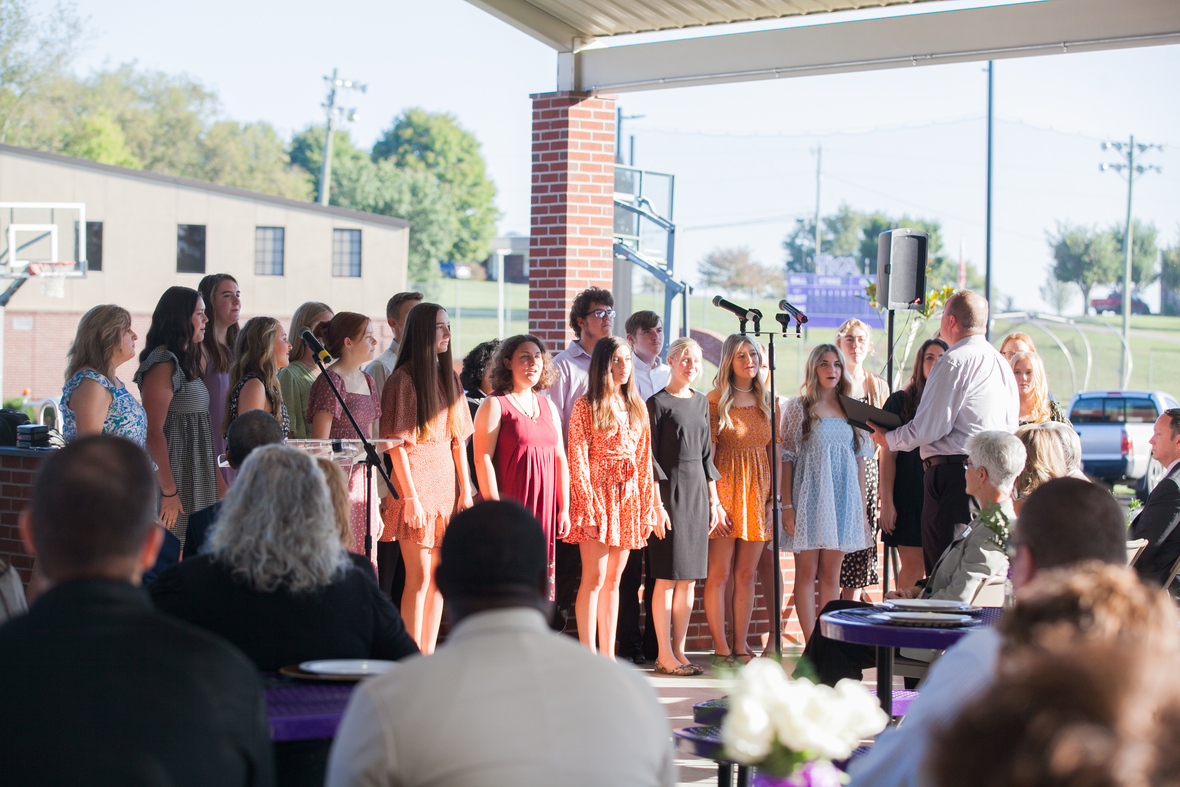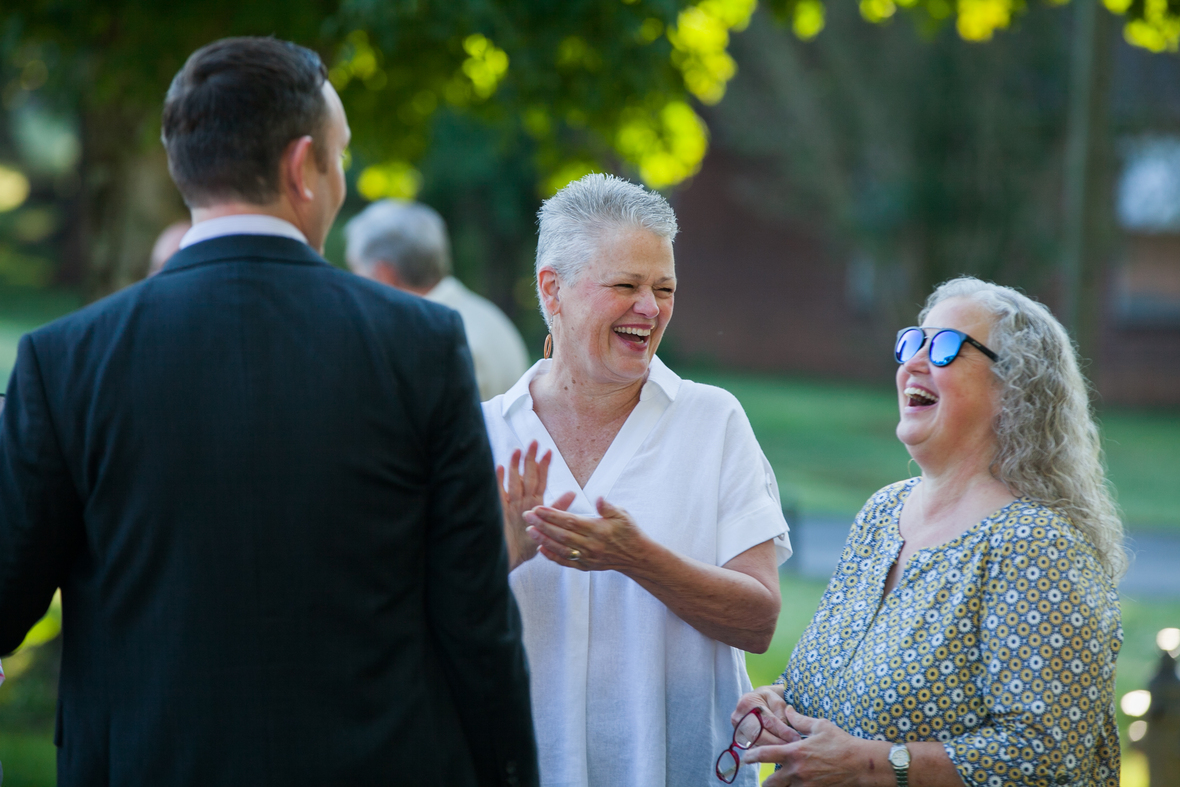# New Website

The King's Academy is ecstatic to announce that our newly updated website has launched! Take a moment and visit thekingsacademy.net to view the full website. Use the link below to view the Alumni page.

On the Alumni page, there are upcoming events, previous editions of the Clarion, alumni newsletters, and a link to update your contact information.

 table div table+table+table+table+table+table+table+table+table+table+table+table+table+table+table+table+table+table+table+table+table+table+table+table div table{width:100%;padding:0}table div table+table+table+table+table+table+table+table+table+table+table+table+table+table+table+table+table+table+table+table+table+table+table+table div table img{width:96.23%;padding:0;float:none}table div table+table+table+table+table+table+table+table+table+table+table+table+table+table+table+table+table+table+table+table+table+table+table+table div table td{width:100%;padding:0 1.88% 18px}/* styles */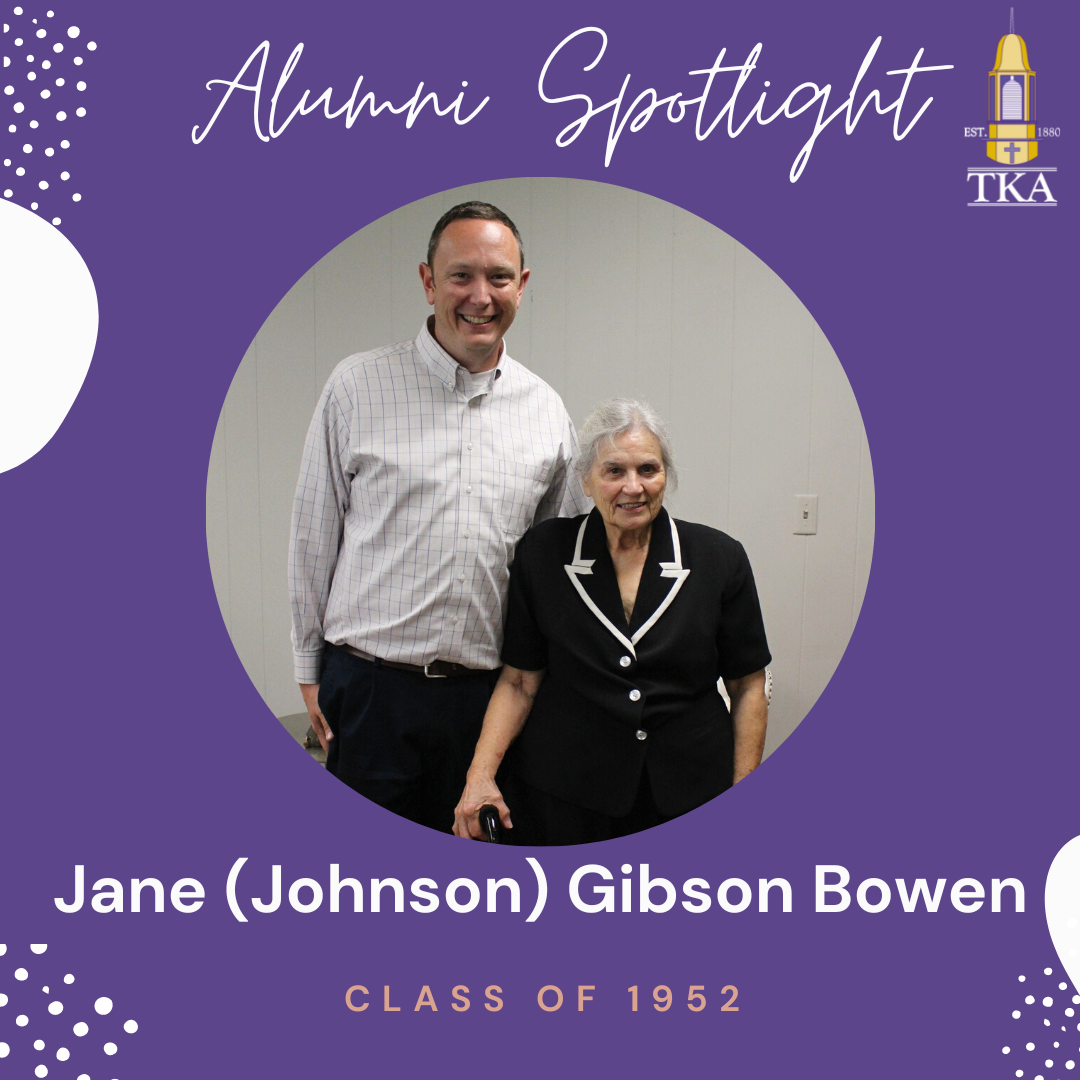# Alumni Spotlight

TKA alumna, Jane Gibson Bowen, graduated with the class of 1952.

We are grateful for the generous gift that was made in memory of Mr. Kim Gibson (Class of 1947) and to his bride, Mrs. Jane Gibson Bowen, who faithfully honored his memory.

 table div table+table+table+table+table+table+table+table+table+table+table+table+table+table+table+table+table+table+table+table+table+table+table+table+table+table div table{width:100%;padding:0}table div table+table+table+table+table+table+table+table+table+table+table+table+table+table+table+table+table+table+table+table+table+table+table+table+table+table div table img{width:96.23%;padding:0;float:none}table div table+table+table+table+table+table+table+table+table+table+table+table+table+table+table+table+table+table+table+table+table+table+table+table+table+table div table td{width:100%;padding:0 1.88% 18px}/* styles */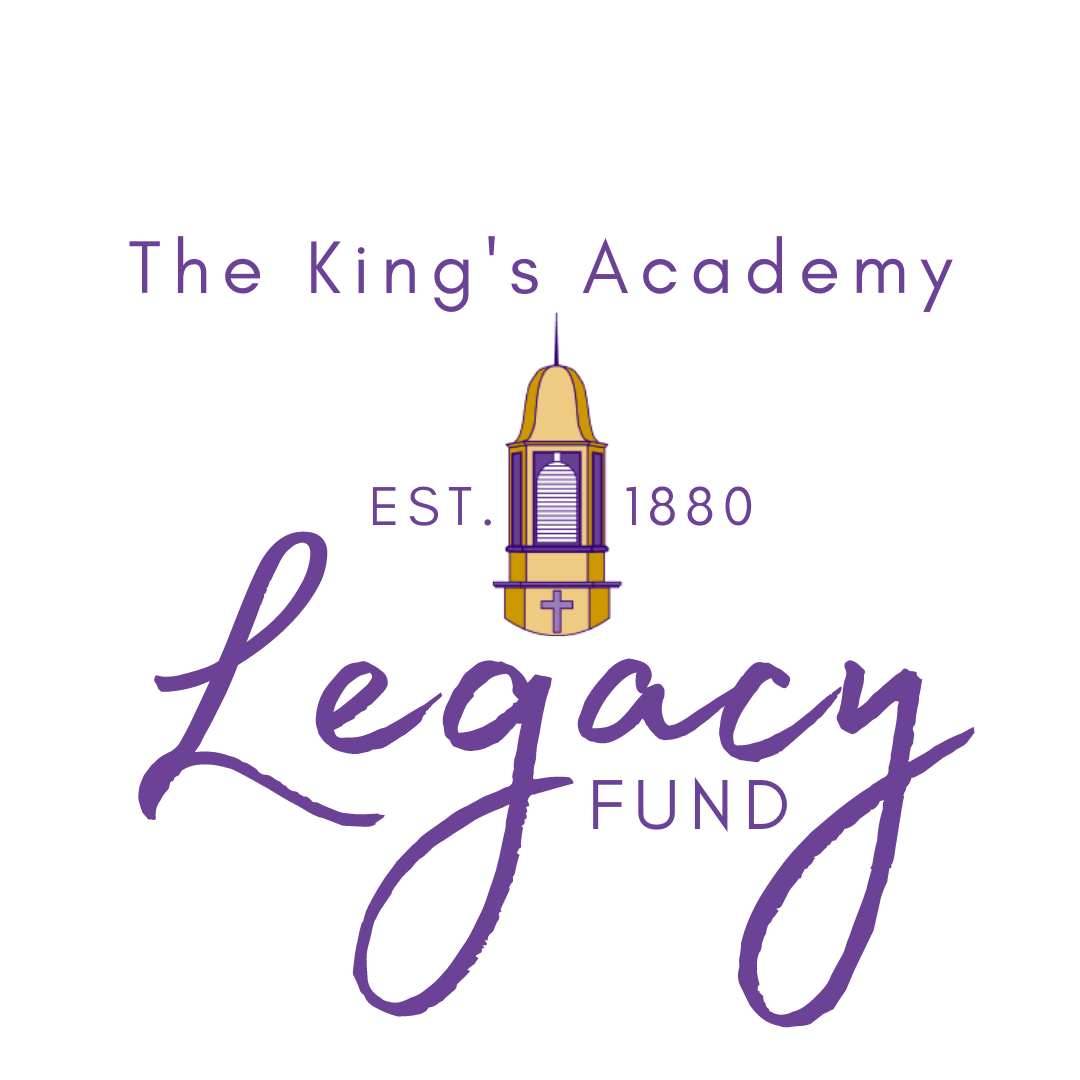# Legacy Fund Update

The Legacy Fund was established in 2021 as a general development fund aimed to allow those willing to leave a legacy of giving at The King's Academy. The 2022 Legacy Fund was well-funded by the generous gifts of many who faithfully gave of their resources.

The Legacy Fund was able to provide the following on campus during the 2021-22 academic year: furnishings for the
Dr. Carroll Reece Reagan Pavilion, Love Where We Live service project needs, bumper stickers and marketing supplies, a new LED sign for the entrance at Boyd's Creek, a new walk-in freezer for the Food Services department, water bottle filling stations throughout campus, bonuses for faculty, mulch for the PreK and Elementary playground, and much more.

We are grateful to all who have given so generously to the Legacy Fund and gratefully welcome all gifts to help further the Legacy of The King's Academy!

 table div table+table+table+table+table+table+table+table+table+table+table+table+table+table+table+table+table+table+table+table+table+table+table+table+table+table+table+table+table div table{width:100%;padding:0}table div table+table+table+table+table+table+table+table+table+table+table+table+table+table+table+table+table+table+table+table+table+table+table+table+table+table+table+table+table div table img{width:96.23%;padding:0;float:none}table div table+table+table+table+table+table+table+table+table+table+table+table+table+table+table+table+table+table+table+table+table+table+table+table+table+table+table+table+table div table td{width:100%;padding:0 1.88% 18px}/* styles */table.module-29{width:74.34%;padding:0}table div table+table+table+table+table+table+table+table+table+table+table+table+table+table+table+table+table+table+table+table+table+table+table+table+table+table+table+table+table+table div table{width:74.34%;float:none;margin-left:auto;margin-right:auto;padding:0}table div table+table+table+table+table+table+table+table+table+table+table+table+table+table+table+table+table+table+table+table+table+table+table+table+table+table+table+table+table+table div table a{border:0 none;text-decoration:none}table div table+table+table+table+table+table+table+table+table+table+table+table+table+table+table+table+table+table+table+table+table+table+table+table+table+table+table+table+table+table div table img{width:100%!important;border:0 none;text-decoration:none}table div table+table+table+table+table+table+table+table+table+table+table+table+table+table+table+table+table+table+table+table+table+table+table+table+table+table+table+table+table+table div table td{width:100%;padding:0}/* styles */
 table div table+table+table+table+table+table+table+table+table+table+table+table+table+table+table+table+table+table+table+table+table+table+table+table+table+table+table+table+table+table+table+table div table{width:100%;padding:0}table div table+table+table+table+table+table+table+table+table+table+table+table+table+table+table+table+table+table+table+table+table+table+table+table+table+table+table+table+table+table+table+table div table img{width:96.23%;padding:0;float:none}table div table+table+table+table+table+table+table+table+table+table+table+table+table+table+table+table+table+table+table+table+table+table+table+table+table+table+table+table+table+table+table+table div table td{width:100%;padding:0 1.88% 18px}/* styles */# Prayer Partners Email

If you would like to receive a monthly email containing detailed prayer requests along with answered prayers please sign up below. Mr. Sandefur sends our "Prayer Partners" email to stakeholders on the first day of each month to those who are willing to intercede on behalf of the Academy. We would value your commitment to pray for the present and future of TKA.

 table div table+table+table+table+table+table+table+table+table+table+table+table+table+table+table+table+table+table+table+table+table+table+table+table+table+table+table+table+table+table+table+table+table+table+table div table{width:100%;padding:0}table div table+table+table+table+table+table+table+table+table+table+table+table+table+table+table+table+table+table+table+table+table+table+table+table+table+table+table+table+table+table+table+table+table+table+table div table img{width:96.23%;padding:0;float:none}table div table+table+table+table+table+table+table+table+table+table+table+table+table+table+table+table+table+table+table+table+table+table+table+table+table+table+table+table+table+table+table+table+table+table+table div table td{width:100%;padding:0 1.88% 18px}/* styles */table div table+table+table+table+table+table+table+table+table+table+table+table+table+table+table+table+table+table+table+table+table+table+table+table+table+table+table+table+table+table+table+table+table+table+table+table+table+table div table{width:100%;padding:0}table div table+table+table+table+table+table+table+table+table+table+table+table+table+table+table+table+table+table+table+table+table+table+table+table+table+table+table+table+table+table+table+table+table+table+table+table+table+table div table img{width:96.23%;padding:0;float:none}table div table+table+table+table+table+table+table+table+table+table+table+table+table+table+table+table+table+table+table+table+table+table+table+table+table+table+table+table+table+table+table+table+table+table+table+table+table+table div table td{width:100%;padding:0 1.88% 18px}/* styles */table div table+table+table+table+table+table+table+table+table+table+table+table+table+table+table+table+table+table+table+table+table+table+table+table+table+table+table+table+table+table+table+table+table+table+table+table+table+table+table+table+table+table+table+table+table div table{width:100%;padding:0}table div table+table+table+table+table+table+table+table+table+table+table+table+table+table+table+table+table+table+table+table+table+table+table+table+table+table+table+table+table+table+table+table+table+table+table+table+table+table+table+table+table+table+table+table+table div table img{width:96.23%;padding:0;float:none}table div table+table+table+table+table+table+table+table+table+table+table+table+table+table+table+table+table+table+table+table+table+table+table+table+table+table+table+table+table+table+table+table+table+table+table+table+table+table+table+table+table+table+table+table+table div table td{width:100%;padding:0 1.88% 18px}/* styles */